# 几何图形的矩阵表示：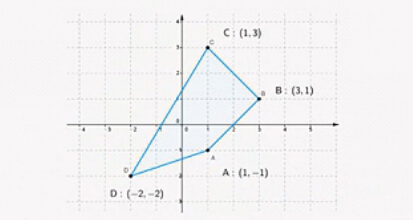P=131211321111

# 平移变换：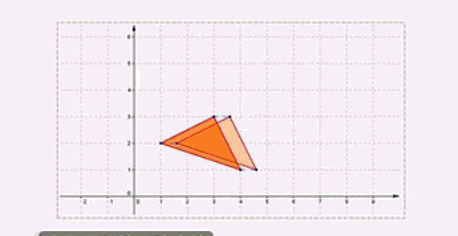#### 例子：

[xy1 ]10a01b001=[x+ay+b1] $\begin{bmatrix} x & y & 1\ \end{bmatrix} \begin{bmatrix} 1 & 0 & 0\\ 0 & 1 & 0\\ a & b & 1\\ \end{bmatrix}= \begin{bmatrix} x+a & y+b & 1\\ \end{bmatrix}$;

# 缩放变换：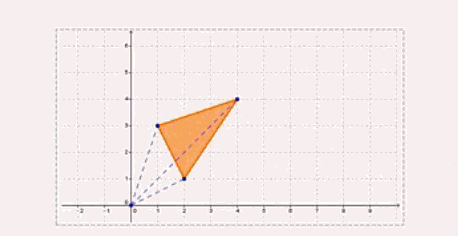#### 例子：

[xy1 ]m000n0001=[mxny1] $\begin{bmatrix} x & y & 1\ \end{bmatrix} \begin{bmatrix} m & 0 & 0\\ 0 & n & 0\\ 0 & 0 & 1\\ \end{bmatrix}= \begin{bmatrix} mx & ny & 1\\ \end{bmatrix}$;

# 旋转变换：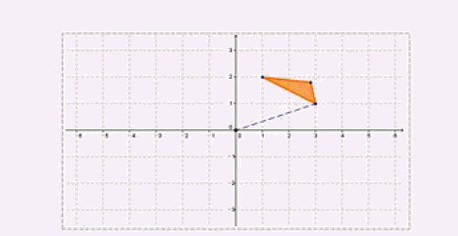#### 例子：

[xy1 ]cosθsinθ0sinθcosθ0001=[xcosθysinθxsinθ+ycosθ1] $\begin{bmatrix} x & y & 1\ \end{bmatrix} \begin{bmatrix} cosθ & sinθ & 0\\ -sinθ & cosθ & 0\\ 0 & 0 & 1\\ \end{bmatrix}= \begin{bmatrix} xcosθ-ysinθ & xsinθ+ycosθ & 1\\ \end{bmatrix}$;

# 对称变换：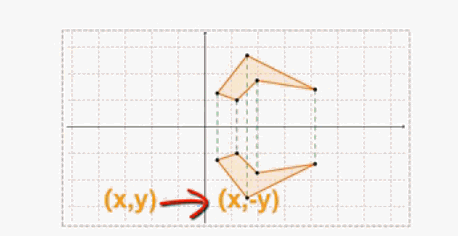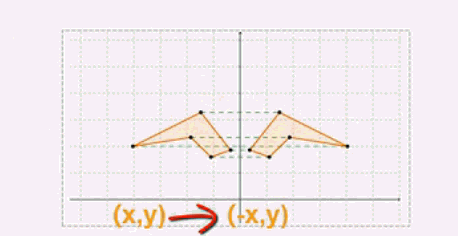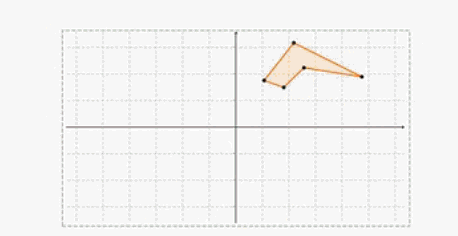# 错切变换：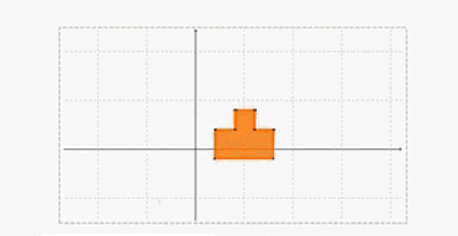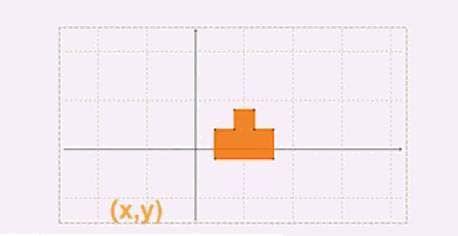c是错切变换因子。

# 组合变换：

### 题目：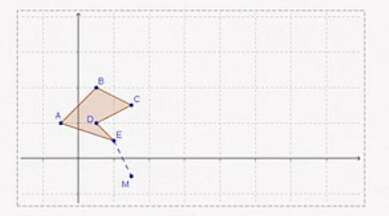### 分析：

（矩阵中的θ是图形绕坐标原点逆时针旋转的角度。）

1. 将M点平移到坐标原点

M(3,-1)，原点O(0,0)，所以平移向量为： MO=(03,0(1))=(3,1) $\vec{MO}=(0-3, 0-(-1))=(-3,1)$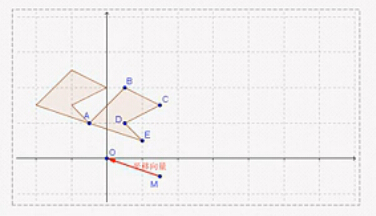2. 将图形绕原点顺时针旋转-90度

（矩阵中的θ是图形绕坐标原点逆时针旋转的角度。）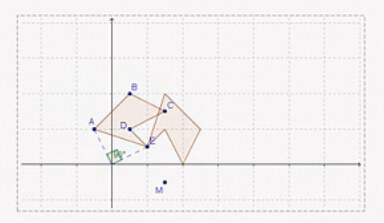3. 以原点为中心，缩放因子为2缩放图形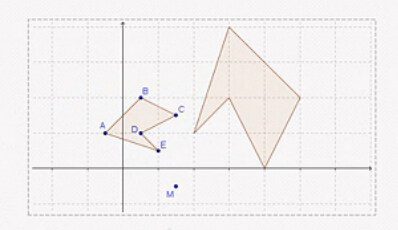4. 将图形反向平移（平移向量为OM，第一步的反向平移）

M(3,-1)，原点O(0,0)，所以平移向量为： OM=(30,(1)0)=(3,1) $\vec{OM}=(3-0, (-1)-0)=(3,-1)$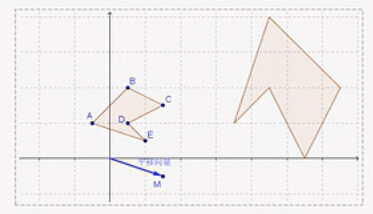### 结果：

P’= P · T $T_{平移}$· T $T_{旋转}$· T $T_{缩放}$· T $T_{平移}$

= 103332111110000103011001cosθsinθ0sinθcosθ0001200020001103011001=91311977313111111 $\begin{pmatrix} -1 & 2 & 1\\ 0 & 1 & 0\\ 3 & -1 & 0\\ 3 & -1 & 0\\ 3 & -1 & 0\\ \end{pmatrix} \begin{pmatrix} 1 & 0 & 0\\ 0 & 1 & 0\\ -3 & 1 & 1\\ \end{pmatrix} \begin{pmatrix} cosθ & -sinθ & 0\\ sinθ & cosθ & 0\\ 0 & 0 & 1\\ \end{pmatrix} \begin{pmatrix} 2 & 0 & 0\\ 0 & 2 & 0\\ 0 & 0 & 1\\ \end{pmatrix} \begin{pmatrix} 1 & 0 & 0\\ 0 & 1 & 0\\ 3 & -1 & 1\\ \end{pmatrix}= \begin{pmatrix} 9 & 7 & 1\\ 13 & 3 & 1\\ 11 & -1 & 1\\ 9 & 3 & 1\\ 7 & 1 & 1\\ \end{pmatrix}$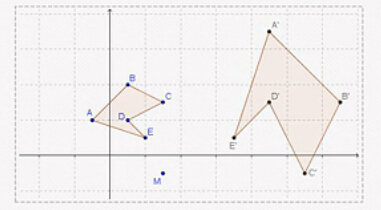# 最后：

09-07
05-24447311-13524
04-14551
10-19
11-22
06-269069
09-0313万+
01-01373
04-171万+